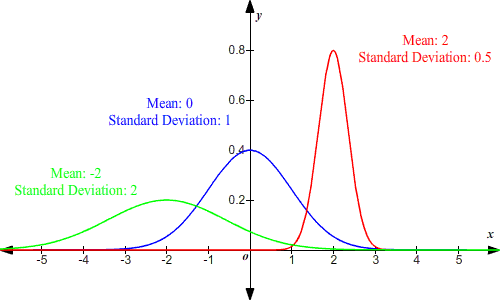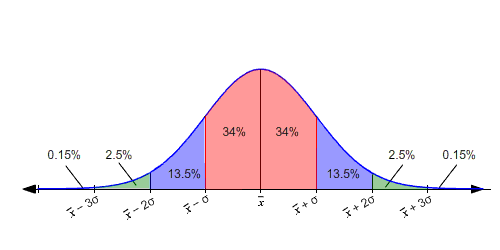# Normal Distribution of Data

A normal distribution is a common probability distribution . It has a shape often referred to as a "bell curve."

Many everyday data sets typically follow a normal distribution: for example, the heights of adult humans, the scores on a test given to a large class, errors in measurements.

The normal distribution is always symmetrical about the mean.

The standard deviation is the measure of how spread out a normally distributed set of data is.  It is a statistic that tells you how closely all of the examples are gathered around the mean in a data set.  The shape of a normal distribution is determined by the mean and the standard deviation. The steeper the bell curve, the smaller the standard deviation.  If the examples are spread far apart, the bell curve will be much flatter, meaning the standard deviation is large.In general, about $68%$ of the area under a normal distribution curve lies within one standard deviation of the mean.

That is, if $\stackrel{¯}{x}$ is the mean and $\sigma$ is the standard deviation of the distribution, then $68%$ of the values fall in the range between $\left(\stackrel{¯}{x}-\sigma \right)$ and $\left(\stackrel{¯}{x}+\sigma \right)$ . In the figure below, this corresponds to the region shaded pink.About $95%$ of the values lie within two standard deviations of the mean, that is, between $\left(\stackrel{¯}{x}-2\sigma \right)$ and $\left(\stackrel{¯}{x}+2\sigma \right)$ .

(In the figure, this is the sum of the pink and blue regions: $34%+34%+13.5%+13.5%=95%$ .)

About $99.7%$ of the values lie within three standard deviations of the mean, that is, between $\left(\stackrel{¯}{x}-3\sigma \right)$ and $\left(\stackrel{¯}{x}+3\sigma \right)$ .

(The pink, blue, and green regions in the figure.)

(Note that these values are approximate.)

Example 1:

A set of data is normally distributed with a mean of $5$ . What percent of the data is less than $5$ ?

A normal distribution is symmetric about the mean. So, half of the data will be less than the mean and half of the data will be greater than the mean.

Therefore, $50%$ percent of the data is less than $5$ .

Example 2:

The life of a fully-charged cell phone battery is normally distributed with a mean of $14$ hours with a standard deviation of $1$ hour. What is the probability that a battery lasts at least $13$ hours?

The mean is $14$ and the standard deviation is $1$ .

$50%$ of the normal distribution lies to the right of the mean, so $50%$ of the time, the battery will last longer than $14$ hours.

The interval from $13$ to $14$ hours represents one standard deviation to the left of the mean. So, about $34%$ of time, the battery will last between $13$ and $14$ hours.

Therefore, the probability that the battery lasts at least $13$ hours is about $34%+50%$ or $0.84$ .

Example 3:

The average weight of a raspberry is $4.4$ gm with a standard deviation of $1.3$ gm. What is the probability that a randomly selected raspberry would weigh at least $3.1$ gm but not more than $7.0$ gm?

The mean is $4.4$ and the standard deviation is $1.3$ .

Note that

$4.4-1.3=3.1$

and

$4.4+2\left(1.3\right)=7.0$

So, the interval $3.1\le x\le 7.0$ is actually between one standard deviation below the mean and $2$ standard deviations above the mean.

In normally distributed data, about $34%$ of the values lie between the mean and one standard deviation below the mean, and $34%$ between the mean and one standard deviation above the mean.

In addition, $13.5%$ of the values lie between the first and second standard deviations above the mean.

Adding the areas, we get $34%+34%+13.5%=81.5%$ .

Therefore, the probability that a randomly selected raspberry will weigh at least $3.1$ gm but not more than $7.0$ gm is $81.5%$ or $0.815$ .

Example 4:

A town has $330,000$ adults. Their heights are normally distributed with a mean of $175$ cm and a variance of $100$ cm $2$ .How many people would you expect to be taller than $205$ cm?

The variance of the data set is given to be $100$ cm $2$ . So, the standard deviation is $\sqrt{100}$ or $10$ cm.

Now, $175+3\left(10\right)=205$ , so the number of people taller than $205$ cm corresponds to the subset of data which lies more than $3$ standard deviations above the mean.

The graph above shows that this represents about $0.15%$ of the data. However, this percentage is approximate, and in this case, we need more precision. The actual percentage, correct to $4$ decimal places, is $0.1318%$ .

$330,000×0.001318\approx 435$

So, there will be about $435$ people in the town taller than $205$ cm.